Latest Banking jobs   »

# Reasoning Ability Quiz For SBI PO/Clerk Mains 2023- 2nd January

Directions (1-5): Consider the character string given below and answer the questions that follow based on certain steps.

C 5 9 U * K & D @ N E / V A 1 Z 2 4 X 7 M # P O S 8 B G ? I

Step I: Each symbol which is immediately preceded by a vowel is placed at the right end of the string such that the first symbol picked from the left end is placed first and the second character is placed immediately after it and so on.
Step II: Each such number which is immediately preceded or followed by a letter which comes before ‘K’ in alphabetical order is/are dropped from the arrangement.
Step III: Each such consonant that is immediately followed a vowel is placed at the left end in the alphabetical order.
Step IV: Each consonant which is immediately preceded and followed by an alphabet is dropped from the arrangement.

Q1. Which of the following element is 7th to the right of the 14th element from the right end of the step IV of the given arrangement?
(a) M
(b) O
(c) 7
(d) #
(e) G

Q2. What is the total number of elements left in the step IV of the given arrangement?
(a) 23
(b) 25
(c) 24
(d) 26
(e) None of these

Q3. How many elements are between ‘&’ and ‘?’ in the step II of the given arrangement?
(a) 18
(b) 16
(c) 14
(d) 20
(e) None of these

Q4. How many such numbers are there in the step IV of the given arrangement which are immediately preceded and followed by an alphabet?
(a) 4
(b) 1
(c) 3
(d) 2
(e) None of these

Q5. What is the sum of all the even numbers which are between ‘P’ and ‘#’ in the step III of the given arrangement?
(a) 8
(b) 6
(c) 12
(d) 10
(e) None of these

Direction (6-9): The question given below consists of two statements numbered I and II given below it. You have to decide whether the data provided in the statements are sufficient to answer the question. Read all the statements and give answer.

Q6. Seven books of different Colours viz. Red, Yellow, Pink, Black, Blue, White and Violet are kept in a stack. The stack is numbered 1-7 such that the stack at the bottom is numbered as 1 and the stack at the top is numbered as 7. Which book is kept at the top of the stack?
Statements:
I. Red book is above the yellow book and only one book is kept between them. Violet book is kept at the bottom of the stack. Only two books are kept between the violet and the yellow book
II. The same number of books are kept above and below the yellow book. Two books are kept between the black and the yellow book. Violet book is kept at the bottom of the stack.
(a) The data in statement I alone are sufficient to answer the question, while the data in statement II alone are not sufficient to answer the question.
(b) The data in statement II alone are sufficient to answer the question, while the data in statement I alone are not sufficient to answer the question.
(c) The data either in statement I alone or in statement II alone are sufficient to answer the question.
(d) The data given in statements I and II together are not sufficient to answer the question.
(e) The data in statements I and II together are necessary to answer the question.

Q7. Four persons P, Q, R and S work in different banks viz. BOB, BOM, IOB, BOI. Their designations are in decreasing order i.e., GM > DGM > AGM > CM. What is S’s designation?
Statements:
I. P works in IOB and is ranked above AGM. CM works in BOI. Neither S nor Q is GM.
II. S works in BOM and is not a GM. R works in BOB. P is a DGM.
(a) The data in statement I alone are sufficient to answer the question, while the data in statement II alone are not sufficient to answer the question.
(b) The data in statement II alone are sufficient to answer the question, while the data in statement I alone are not sufficient to answer the question.
(c) The data either in statement I alone or in statement II alone are sufficient to answer the question.
(d) The data given in statements I and II together are not sufficient to answer the question.
(e) The data in statements I and II together are necessary to answer the question.

Q8. Eight people- M, N, O, P, Q, R, S and T are living in a different storey building but not necessarily in the same order. The lowest floor is numbered as 1, the floor just above that is numbered as 2 and so on up to the topmost floor is numbered as 8. Who among the following lives on the topmost floor?
Statements:
I. O lives on the lowermost floor. Not more than three people live above P. R lives on an even numbered floor immediately below N. Two people live between S and T who lives below S. Neither P nor S lives on the topmost floor.
II. Q lives on an odd numbered floor immediately below M. N lives on the 3rd floor. T lives on an even numbered floor below S. R is adjacent to O who lives on the lowermost.
(a) The data in statement I alone are sufficient to answer the question, while the data in statement II alone are not sufficient to answer the question.
(b) The data in statement II alone are sufficient to answer the question, while the data in statement I alone are not sufficient to answer the question.
(c) The data either in statement I alone or in statement II alone are sufficient to answer the question.
(d) The data given in statements I and II together are not sufficient to answer the question.
(e) The data in statements I and II together are necessary to answer the question.

Q9. Ten people A, B, C, D, E, F, G, H, I, and J are seated around a rectangular table and all are facing inside, with one at each corner and with one at each shorter side of the table and with two at each longer sides of the table. D who sits at one of the shorter sides faces J. What is the position of E with respect to A?
Statements:
I. A who sits immediate right of D sits third to the left of B. G sits third to the left of H who faces B. C faces E who is an immediate neighbour of I.
II. H who sits immediate left of D sits second to the right of C. B sits third to the right of A who sits second to the right of H. G who faces A sits second to the left of F.
(a) The data in statement I alone are sufficient to answer the question, while the data in statement II alone are not sufficient to answer the question.
(b) The data in statement II alone are sufficient to answer the question, while the data in statement I alone are not sufficient to answer the question.
(c) The data either in statement I alone or in statement II alone are sufficient to answer the question.
(d) The data given in statements I and II together are not sufficient to answer the question.
(e) The data in statements I and II together are necessary to answer the question.

Direction (10-12): The question given below consists of two statements numbered I and II given below it. You have to decide whether the data provided in the statements are sufficient to answer the question. Read all the statements and give answer.

Q10. Six teams I, C, U, T, J, and K competed in Commonwealth Games and secured different number of Gold medals. Which team has secured the 3rd highest number of Gold medals?
Statements:
I. C secured more medals than T which secured more medals than U. Only I secured more medals than K. J secured more medals than T.
II. T secured more medals than only U. J secured more medals than only two teams. K secured more medals than C.
(a) The data in statement I alone are sufficient to answer the question, while the data in statement II alone are not sufficient to answer the question.
(b) The data in statement II alone are sufficient to answer the question, while the data in statement I alone are not sufficient to answer the question.
(c) The data either in statement I alone or in statement II alone are sufficient to answer the question.
(d) The data given in statements I and II together are not sufficient to answer the question.
(e) The data in statements I and II together are necessary to answer the question.

Q11. Six persons A, B, C, D, E, and F sit around a circular table equidistant from each other such that all persons face towards the center of the table but not necessarily in the same order. Who sits immediate left of D?
Statements:
I. F sits immediate left of B. Two persons sit between A and E. C doesn’t sit opposite to F.
II. Two persons sit between B and C. One person sits between C and F from either side. D sits opposite to F. A doesn’t sit adjacent to C.
(a) The data in statement I alone are sufficient to answer the question, while the data in statement II alone are not sufficient to answer the question.
(b) The data in statement II alone are sufficient to answer the question, while the data in statement I alone are not sufficient to answer the question.
(c) The data either in statement I alone or in statement II alone are sufficient to answer the question.
(d) The data given in statements I and II together are not sufficient to answer the question.
(e) The data in statements I and II together are necessary to answer the question.

Q12. Six persons (A, B, C, D, E and F) live on different floors of 6-storey building such that bottommost floor is numbered as 1, floor just above it is numbered as 2 and so on. Who lives on the topmost floor?
Statements:
I. E who lives immediately above D lives three floors below B. A lives immediately above C.
II. C who lives two floors below B lives two floors above D. A who doesn’t live on the topmost floor lives above E. D doesn’t live on 2nd floor.
(a) The data in statement I alone are sufficient to answer the question, while the data in statement II alone are not sufficient to answer the question.
(b) The data in statement II alone are sufficient to answer the question, while the data in statement I alone are not sufficient to answer the question.
(c) The data either in statement I alone or in statement II alone are sufficient to answer the question.
(d) The data given in statements I and II together are not sufficient to answer the question.
(e) The data in statements I and II together are necessary to answer the question.

Directions (13-15): In each of the questions given below, a group of letters is given followed by some combinations of numbers/symbols. You have to find out which of the given combination correctly represents the group of letters based on the numbers/symbols codes and the conditions given below. If none of the given combinations represents the group of letters correctly, give (e) i.e., none of these as the answer.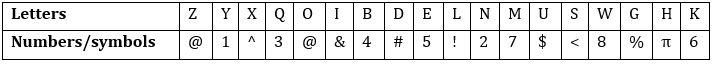Condition for coding the group letters:
(i) If the first letter is a vowel and the last letter is a consonant, the codes for the first and the last letter are to be interchanged.
(ii) If the first as well as the last letter is a consonant, then both are to be coded by the code for the third letter of the word.
(iii) If the first as well as the last letter is vowel, then both are to be coded by the code for the last letter.
(iv) If the first letter is a consonant and the last letter is a vowel, the codes for the first and the last letter are to be coded as β.

Q13. What is the code for the word ‘BELDUW’?
(a) 45!#\$8
(b) !5!#\$!
(c) !!5#\$!
(d) 85!#\$4
(e) None of these

Q14. What is the code for the word ‘NGZKHE’?
(a) 2%@6 π5
(b) β%@6 β π
(c) 2@6% π5
(d) β@6@ β π
(e) None of these

Q15. What is the code for the word ‘ISKONE’?
(a) 5<6@25
(b) &<6@25
(c) 56<@25
(d) 5<6@2&
(e) None of these

Solutions

Solution (1-5):
Given series – C 5 9 U * K & D @ N E / V A 1 Z 2 4 X 7 M # P O S 8 B G ? I
Step I – C 5 9 U K & D @ N E V A 1 Z 2 4 X 7 M # P O S 8 B G ? I */
Step II – C 9 U K & D @ N E V A Z 2 4 X 7 M # P O S B G ? I * /
Step III – N P V C 9 U K & D @ E A Z 2 4 X 7 M # O S B G ? I * /
Step IV – N C 9 U K & D @ E A Z 2 4 X 7 M # O G ? I * /

S1. Ans. (d)
S2. Ans. (a)
S3. Ans. (a)
S4. Ans. (d)
S5. Ans. (b)

S6. Ans. (b)
Sol. From statement II: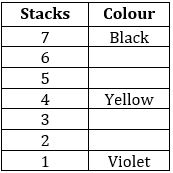Hence, the data in statement II alone are sufficient to answer the question, while the data in statement I alone are not sufficient to answer the question.

S7. Ans. I
Sol. From statement I and II together: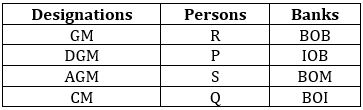Hence, the data in statements I and II together are necessary to answer the question.

S8. Ans. (d)
Sol. From the given statements, we can’t determine the answer because of lack information. Hence, the data given in statements I and II together are not sufficient to answer the question.

S9. Ans. (e)
Sol. From statement I and II together: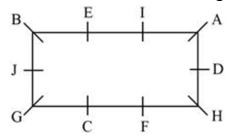Hence, the data in statements I and II together are necessary to answer the question.

S10. Ans. (e)
Sol. From statement I and II together: I > K > C > J > T > U
Hence, the data in statements I and II together are necessary to answer the question.

S11. Ans. (d)
Sol. From the given statements, we can’t determine the answer because of lack information. Hence, the data given in statements I and II together are not sufficient to answer the question.

S12. Ans. (b)
Sol. From statement II alone: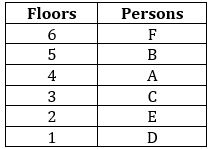Hence, the data in statement II alone are sufficient to answer the question, while the data in statement I alone are not sufficient to answer the question.

S13. Ans. (b)
Sol. Condition (ii) is applied.

S14. Ans. (e)
Sol. Condition (iv) is applied.

S15. Ans. (a)
Sol. Condition (iii) is applied.#### Congratulations!